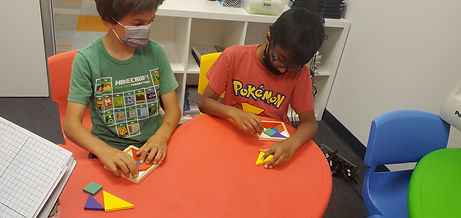## Mr. Jake

### Target 1​

###### Lesson Type:

New

Geometry

:

Modeling

Visualize spatial relationships between shapes and planar space.

###### 1:

Analyze the fractional relationships among tangram shapes.

###### 2:

Solve simple tangram puzzles by recognizing which spaces in the puzzle the given seven shapes can and cannot fit.

###### 3:

Recognize and apply reflections (flips), translations (slides), and rotations (turns).

6th

###### Vocabulary:

Tangram, Shape, Polygon, Fraction

Activities:

Solved puzzles using tangrams

Determined how each tangram piece is related and how they can be used to make one another### Home Exploration

###### Guiding Questions:## Absent Students:

### Target 2

:

###### 1:

Understand that there are multiple ways to divide multi-digit numbers.

###### 2:

Understand the role of place-value in division strategies.

###### 3:

Use the standard algorithm for solving multi-digit division equations.

###### 4:

Understand that there can be a remainder in a division problem.

5th

###### Vocabulary:

Division, Divisor, Dividend, Quotient

Activities:

Practiced solving division problems

Used standard division and long division techniques to solve division problems### Home Exploration

###### Guiding Questions:### Target 3

:

###### Vocabulary:

Activities:### Home Exploration